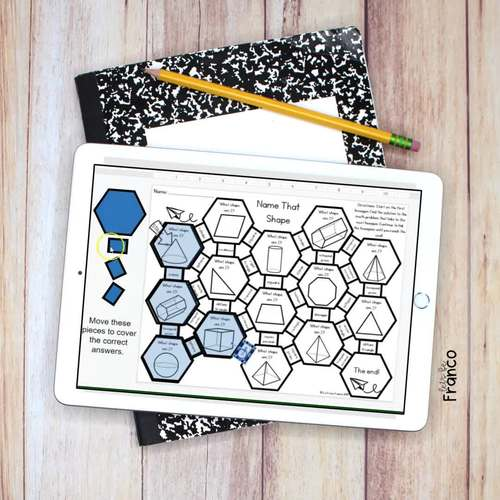# Math Maze Digital Puzzle Reviews 5th Grade Math Station

Rated 4.87 out of 5, based on 45 reviews
45 Ratings;
4th - 6th
Subjects
Resource Type
Standards
Formats Included
• PDF
Pages
50+
\$12.00
\$12.00
Report this resource to TPT

#### What educators are saying

My students love the maze format! They are highly motivated to solve the problems and get to “the end”
Intended to use them for e-learning days, but came in handy to print and use when our school network went down.

### Description

Do you want to add mazes and puzzles to your math workshop? These mazes are a perfect addition to any math class. Students will work through the maze by solving 5th grade math problems. The goal of the mazes is to review computation in an engaging way!

NOW INCLUDES a Google Slides version for distance learning!

-27 Different Mazes

- Google Slides interactive version of the mazes

All the puzzles that are included:

- Direction Cards

- Multiplication Facts

- Decimal Rounding

- Order of Operations

- Single Digit Multiplication

- Double Digit Multiplication

- Single Digit Divisor Division

- Double Digit Divisor Division

- Multiplication and Division

- Multiplying with Powers of Ten

- Comparing Decimals

- Decimal Subtraction

- Decimal Multiplication

- Decimal Division

- Decimal Multiplication and Division

- Fraction Subtraction

- Fraction Multiplication

- Fraction Division

- Fraction Multiplication and Division

- Convert Time

- Convert Measurement – Customary

- Convert Measurement – Metric

- Name that Shape

- Volume of Rectangular Prisms

Other products you might like:

Pumpkin Whole Number Multiplication Task Cards

Multiply Decimal Snowman Escape the Classroom

5th Grade Math Escape Activities Bundle

Math and Science Bulletin Boards

Math Activities

Are you using one of my products? Post one on Instagram and tag me @letsbefranco so I can share!

If you would like to see my products in action, check out my blog Let’s be Franco or follow me on Pinterest Let’s be Franco Pinterest

** How to get TPT credit to use on future purchases **

Go to your My Purchases page. Beside each purchase, you will see a “Provide Feedback” button. Click it and give a rating and leave a comment! You will have credits to use on future purchases.

** Be the first to hear about my new products and discounts! **

Let me know what you think!

Happy Teaching!

Total Pages
50+
Included
Teaching Duration
N/A
Report this resource to TPT
Reported resources will be reviewed by our team. Report this resource to let us know if this resource violates TPT’s content guidelines.

### Standards

to see state-specific standards (only available in the US).
Know relative sizes of measurement units within one system of units including km, m, cm; kg, g; lb, oz.; l, ml; hr, min, sec. Within a single system of measurement, express measurements in a larger unit in terms of a smaller unit. Record measurement equivalents in a two-column table. For example, know that 1 ft is 12 times as long as 1 in. Express the length of a 4 ft snake as 48 in. Generate a conversion table for feet and inches listing the number pairs (1, 12), (2, 24), (3, 36),...
Use the four operations to solve word problems involving distances, intervals of time, liquid volumes, masses of objects, and money, including problems involving simple fractions or decimals, and problems that require expressing measurements given in a larger unit in terms of a smaller unit. Represent measurement quantities using diagrams such as number line diagrams that feature a measurement scale.
Apply the area and perimeter formulas for rectangles in real world and mathematical problems. For example, find the width of a rectangular room given the area of the flooring and the length, by viewing the area formula as a multiplication equation with an unknown factor.
Recognize angles as geometric shapes that are formed wherever two rays share a common endpoint, and understand concepts of angle measurement:
Recognize angle measure as additive. When an angle is decomposed into non-overlapping parts, the angle measure of the whole is the sum of the angle measures of the parts. Solve addition and subtraction problems to find unknown angles on a diagram in real world and mathematical problems, e.g., by using an equation with a symbol for the unknown angle measure.